## UV index and UV dose: details of the calculation data version 2.x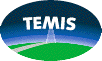monitoring:

UV index
UV dose

Main data product description page

### Calculation of the UV index and UV dose

Relevant for the biological effect of UV radiation is the UV spectrum at the Earths surface weighted by the action spectrum. The following graph illustrates this for the effect of erythema. The UV spectrum at the surface (red solid line) is multiplied by the erythemal action spectrum (blue dashed line), giving the "weighted UV spectrum" (black dotted line).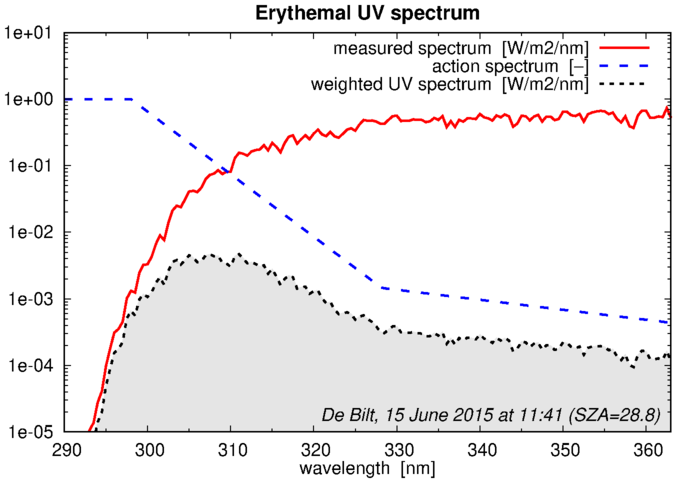Integration of the "weighted UV spectrum" over all wavelengths up to 400 nm, i.e. the surface below the black-dotted line, is the so-called "UV index", in W/m2, at the moment of measurement of the UV spectrum, which we will denote here by UVW(t). This UVW(t) depends essentially on the amount of ozone (TO) and the position of the sun (expressed in terms of the solar zenith angle, SZA).

Many UV spectra measured at the groundstations of De Bilt (The Netherlands) and Paramaribo (Suriname) have been used to determine a parametrisation of UVW(t) as function of TO and SZA:

```      UVW(t) = UVW{TO,SZA}
```
where `{...}` refers to the parametrisation: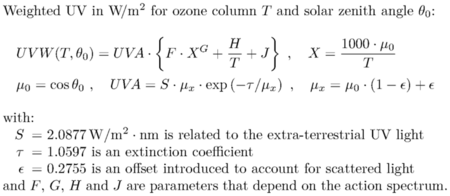Function and fit approach are described by Allaart et al. (Meteorological Applications 11, 59-65, 2004). With the version v2.0 major upgrade in 2017, the fits have been done again using more ground-based measurements from De Bilt and Paramaribo, which has led to new values for S, τ, ε and the four parameters associated with a given action spectrum. Differences in, for example, the UVA part of the equation are less than 10% for SZAs below 80°.

The basis of this parametrisation is described by Allaart et al. (2004) using the erythemal action spectrum. For the TEMIS v2.x data the parametrisation is determined anew, using on more groundbased UV measurements and the latest version of the three action spectra. The current fit parameters are as follows (where `E` is discussed below):

 action spectrum S τ ε F G H J E Erythemal 2.0877 1.0597 0.2755 0.0477 1.6325 5.6499 0.0485 0.12 Vitamin-D 0.1101 1.6481 7.0745 0.0 0.19 DNA-damge 0.0137 2.4564 3.3694 0.0 0.11

The parametrisation thus provides a "first guess" of the UV dose rate in W/m2 at a given moment. For the TEMIS UV index and UV dose data service this first guess is corrected for a number of effects:

```       UVR(t) = UVW{TO,SZA} * f(D) * f(A) * f(H) * f(C)
```
with corrections for (some details are given on separate pages):
```  f(D) = varying Sun-Earth distance
f(A) = surface albedo
f(H) = surface elevation
f(C) = presence of clouds
```
where the first three corrections are assumed constant during a given day, while the presence of clouds may vary during the day. There is no explicit correction for the presence of aerosols; see this page for some notes on that issue.

### Erythemal UV index data product

The erythemal UV index (UVI), as communicated to the public to raise awareness of possible harmfull effects of UV radiation, is determined for "local solar noon" (LSN), i.e. the moment when the Sun is highest in the sky, in the absence of clouds:
```       UVR(t=LSN) = UVW{TO(t=LSN),SZA(t=LSN)} * f(D) * f(A) * f(H)

     UVI = UVR(t=LSN) / 0.025
```
The latter equation is used because the UVI is usually given -- and therefore also via the TEMIS UV index data service -- in dimensionless units, where 1 unit corresponds to 25 mW/m2.

Note that in principle one could also provide a UV index for the other action spectra, but this is not done to avoid confustion in the communication: the UVI always refers to the clear-sky erythemal UV index.

### UV dose data products

The UV dose (UVD) is the total daily amount of UV radiation for a given biological effect. The TEMIS UV dose data service provides the UV dose for three action spectra: erythemal, vitamin-D and DNA-damage, both using clear-sky conditions and with a correction for the presence of clouds.

For the TEMIS UV dose we assume that the total ozone column is constant throughout the day at the local solar noon value:

```       UVR(t) = UV{TO(t=LSN),SZA(t)} * f(D) * f(A) * f(H) * f(C(t))

     UVD = ∫ UVR(t) * dt
```
The UV dose at moment t, the so-called UV dose rate (UVR, which is in W/m2), is integrated over the day with a time step of 5 minutes, where for the clear-sky version the last term is omitted. The daily UV dose UVD is then given in kJ/m2.

Since there is already some UV radiation some time before sunrise and some time after sunset (sunrise and sunset have SZA = 90 degrees), due to scattering of UV radiation in the atmosphere, we perform the integration over the period between what we have termed scatterrise and scatterset (some 1.5 hours extra on either side).

The following graph gives an example of the UV dose rate, both cloud-modified (red solid line; day integral = 3.06 kJ/m2) and clear-sky (blue dashed line; day integral = 4.07 kJ/m2) for 16 June 2012 over De Bilt (The Netherlands) as function of time.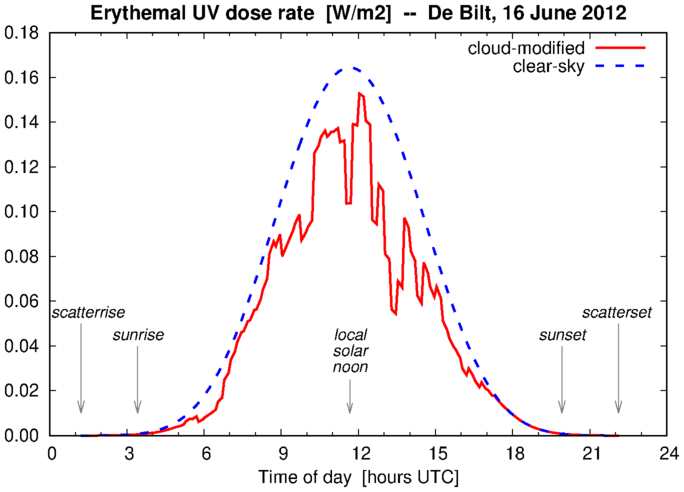### Error estimate for the UV data products

The TEMIS UV index and UV dose data products come with an estimate of the error, i.e. the uncertainty, which is derived from the uncertainties in the input of the Eqs. `` and ``.

 `UVW` uncertainty from the parametrisation, estimated to be `0.0001 + (E*cos(SZA))^2` where `E` is a fit parameter that depends on the action spectrum (see table above) `TO  ` uncertainty from the total ozone data product `f(D)` no uncertainty; we assume the varying Sun-Earth distance to be well-known `f(A)` uncertainty from the albedo data product `f(H)` standard deviation from the averaging cell of the surface elevation `f(C)` estimated when formulating the cloud modification factor

These error terms are essentially independent from one another and are therefore added quadratically.

### Local solar time vs. UTC

The integration of Eq. `` is performed in terms of local solar time (LST), with 12h local solar noon (LSN), i.e. the moment when the Sun is highest in the sky. The begin and end point of the integration, scatterrise and scatterset, lie symmetric around LSN.

Conversion of the `t_LST` to regular `t_UTC` is needed, e.g. for the ingestion of the cloud data and for comparisons against groundbased measurements. A rough estimate of the conversion is based on longitude (1 hour per 15 degrees). But we need a more accurate conversion, one that accounts for the fact that the Earths orbit around the Sun is an ellipse rather than a circle: we need to use the so-called "equation of time" in the conversion:

```       t_UTC = t_LST − lon / 15.0 − eqtime
```
with longitude (`lon`) in degrees and the times in fractional hours. The equation of time (`eqtime`) depends on the day of the year and the t_LST and can be up to 15 minutes.

The definition `eqtime` can be found, e.g., on this external web page. The equation given on that webpage is not exactly accurate, e.g. because it is based a year of 365 days, leading to differences of 10 seconds or less. But this is not a problem given the other uncertainties in the UV data processing.

References:

Allaart, M., Van Weele, M., Fortuin P. and Kelder, H.: 2004,
An Empirical model to predict the UV-index based on solar zenith angle and total ozone
Meteorological Applications (Royal Met. Society), 11, 59-65.
doi:10.1017/S1350482703001130

Zempila, M.M., Van Geffen, J.H.G.M., Taylor, M., Fountoulakis, I., Koukouli, M.E., Van Weele, M., Van der A, R.J., Bais, A., Meleti, C. and Balis, D.: 2017,
TEMIS UV product validation using NILU-UV ground-based measurements in Thessaloniki, Greece
Atmos. Chem. Phys. 17, 7157-7174.
doi:10.5194/acp-17-7157-2017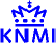last modified: 30 January 2023
data product contact: Jos van Geffen & Michiel van Weele & Ronald van der A